# Linear Dispersion Relation

Let us write [see Equation (5.33)]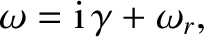(6.1)

where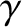is the growth-rate of the tearing mode, whereas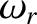is the real frequency of the mode in the laboratory frame. We shall assume that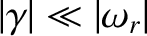. This assumption can easily be verified a posteriori. (See Table 6.2.) Asymptotic matching (see Section 3.8) between the tearing mode solution in the outer region and that in the thin resistive layer, surrounding the rational surface (see Section 3.7), that constitutes the inner region, yields a linear tearing mode dispersion relation of the form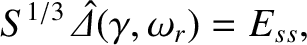(6.2)

where use has been made of Equations (3.74) and (5.70). Here,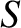is the Lundquist number at the rational surface [see Equation (5.48)],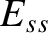is the real tearing stability index (see Section 3.8), whereas the complex layer matching parameter,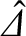, is defined in Equation (5.69).

In a conventional tokamak fusion reactor [assuming that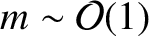, where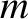is the poloidal mode number of the tearing perturbation],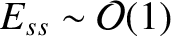,6.1 and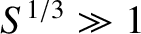. (See Table 1.5.) Hence, the previous dispersion relation can only be satisfied iftakes a value that renders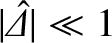. It is clear from Equations (5.61)–(5.65), (5.93), (5.94), (5.96), and (5.98) that this goal can be achieved in all of the constant-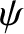linear response regimes if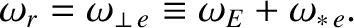(6.3)

Here,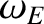and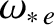are the E-cross-B and electron diamagnetic frequencies, respectively, at the rational surface. [See Equations (5.21), (5.29), (5.44), and (5.45).] The previous equation implies that the tearing mode co-rotates with the electron fluid at the rational surface . (See Section 2.24.)